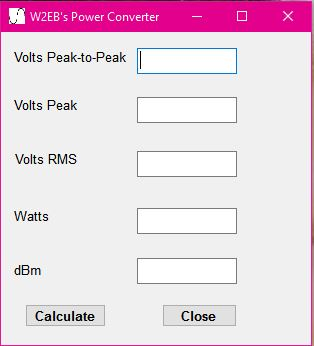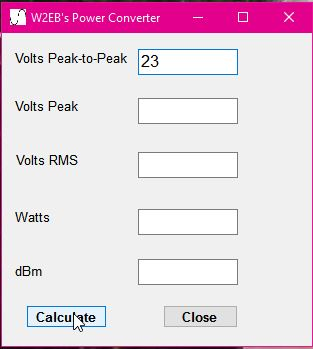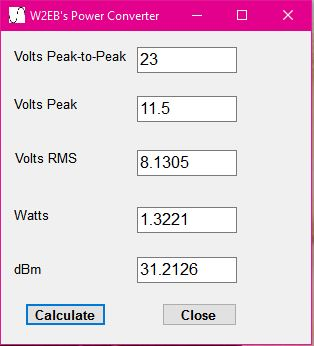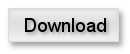# A Simple RF Power Units CalculatorI often find a need to convert between units when working with RF circuits or software.  For example, when testing a Power amp with an oscilloscope: what power does XXX volts peak to peak equal?  This calculator is a simple utility to calculate any of the other 4 units when you enter one.Operation

Operation is utter simplicity:  simply enter what you know and click "Calculate".  The calculator will populate the remaining unknown units.-Bill Lazure